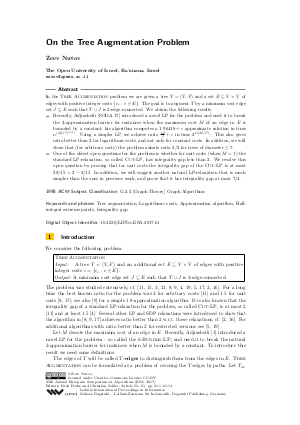Document# On the Tree Augmentation Problem

### Author Zeev Nutov## File

LIPIcs.ESA.2017.61.pdf
• Filesize: 0.53 MB
• 14 pages

## Cite As

Zeev Nutov. On the Tree Augmentation Problem. In 25th Annual European Symposium on Algorithms (ESA 2017). Leibniz International Proceedings in Informatics (LIPIcs), Volume 87, pp. 61:1-61:14, Schloss Dagstuhl - Leibniz-Zentrum für Informatik (2017)
https://doi.org/10.4230/LIPIcs.ESA.2017.61

## Abstract

In the Tree Augmentation problem we are given a tree T=(V,F) and a set E of edges with positive integer costs {c_e:e in E}. The goal is to augment T by a minimum cost edge set J subseteq E such that T cup J is 2-edge-connected. We obtain the following results. Recently, Adjiashvili [SODA 17] introduced a novel LP for the problem and used it to break the 2-approximation barrier for instances when the maximum cost M of an edge in E is bounded by a constant; his algorithm computes a 1.96418+epsilon approximate solution in time n^{{(M/epsilon^2)}^{O(1)}}. Using a simpler LP, we achieve ratio 12/7+epsilon in time ^{O(M/epsilon^2)}. This also gives ratio better than 2 for logarithmic costs, and not only for constant costs. In addition, we will show that (for arbitrary costs) the problem admits ratio 3/2 for trees of diameter <= 7. One of the oldest open questions for the problem is whether for unit costs (when M=1) the standard LP-relaxation, so called Cut-LP, has integrality gap less than 2. We resolve this open question by proving that for unit costs the integrality gap of the Cut-LP is at most 28/15=2-2/15. In addition, we will suggest another natural LP-relaxation that is much simpler than the ones in previous work, and prove that it has integrality gap at most 7/4.
##### Keywords
• Tree augmentation
• Logarithmic costs
• Approximation algorithm
• Half-integral extreme points
• Integrality gap

## Metrics

• Access Statistics
• Total Accesses (updated on a weekly basis)
0

## References

1. D. Adjiashvili. Beating approximation factor two for weighted tree augmentation with bounded costs. In SODA, pages 2384-2399, 2017.2. J. Cheriyan and Z. Gao. Approximating (unweighted) tree augmentation via lift-and-project, part II. Manuscript, 2015.3. J. Cheriyan, T. Jordán, and R. Ravi. On 2-coverings and 2-packing of laminar families. In ESA, pages 510-520, 1999.4. J. Cheriyan, H. Karloff, R. Khandekar, and J. Koenemann. On the integrality ratio for tree augmentation. Operation Research Letters, 36(4):399-401, 2008.5. N. Cohen and Z. Nutov. A (1+ln2)-approximation algorithm for minimum-cost 2-edge-connectivity augmentation of trees with constant radius. Theoretical Computer Science, 489-490:67-74, 2013.6. M. Cygan. Private communication, 2016.7. M. Cygan, F. Fomin, F. Kowalik, D. Lokshtanov, D. Marx, M. Pilipczuk, M. Pilipczuk, and S. Saurabh. Parameterized Algorithms. Springer, 2016.8. G. Even, J. Feldman, G. Kortsarz, and Z. Nutov. A 3/2-approximation for augmenting a connected graph into a two-connected graph. In APPROX, pages 90-101, 2001.9. G. Even, J. Feldman, G. Kortsarz, and Z. Nutov. A 1.8-approximation algorithm for augmenting edge-connectivity of a graph from 1 to 2. ACM Transactions on Algorithms, 5(2), 2009.10. S. Fiorini, M. Groß, J.Könemann, and L. Sanitá. A 3/2-approximation algorithm for tree augmentation via chvátal-gomory cuts. https://arxiv.org/abs/1702.05567, Feb 27, 2017.
11. G. Frederickson and J. Jájá. Approximation algorithms for several graph augmentation problems. SIAM J. Computing, 10:270-283, 1981.12. M. Goemans, A. Goldberg, S. Plotkin, E. Tardos D. Shmoys, and D. Williamson. Improved approximation algorithms for network design problems. In SODA, pages 223-232, 1994.13. D. Hochbaum, N. Megiddo, J. Naor, and A. Tamir. Tight bounds and 2-approximation algorithms for integer programs with two variables per inequality. Math. Programming, 62:69–83, 1993.14. K. Jain. A factor 2 approximation algorithm for the generalized steiner network problem. Combinatorica, 21(1):39-60, 2001.15. S. Khuller and R. Thurimella. Approximation algorithms for graph augmentation. J. of Algorithms, 14:214-225, 1993.16. G. Kortsarz and Z. Nutov. LP-relaxations for tree augmentation. In APPROX-RANDOM, pages 13:1-13:16, 2016.17. G. Kortsarz and Z. Nutov. A simplified 1.5-approximation algorithm for augmenting edge-connectivity of a graph from 1 to 2. ACM Transactions on Algorithms, 12(2):23, 2016.18. L. C. Lau, R. Ravi, and M. Singh. Iterative Methods in Combinatorial Optimization. Cambridge University Press, 2011.19. Y. Maduel and Z. Nutov. Covering a laminar family by leaf to leaf links. Discrete Applied Mathematics, 158(13):1424-1432, 2010.20. D. Marx and L. Végh. Fixed-parameter algorithms for minimum-cost edge-connectivity augmentation. ACM Transactions on Algorithms, 11(4):27, 2015.21. H. Nagamochi. An approximation for finding a smallest 2-edge connected subgraph containing a specified spanning tree. Discrete Applied Mathematics, 126:83-113, 2003.X

Feedback for Dagstuhl Publishing